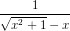# Question & Answer: Create a Java program called RoundingError…..

Create a Java program called RoundingError

(a) Using your Java program, evaluate f(x) =for x = 108 and give the answer provided by the program. Do you believe this is the correct answer? Why or why not?

Don't use plagiarized sources. Get Your Custom Essay on
Question & Answer: Create a Java program called RoundingError…..
GET AN ESSAY WRITTEN FOR YOU FROM AS LOW AS \$13/PAGE

(b) Rationalize the denominator of f(x) to get g(x). Using your Java program, evaluate g(x) for x = 108 and give the answer provided by the program. do you believe this is the correct answer? why or why not?

(b) Rationalizing 1/(x2+1) -x

Multiplying numerator and denominator by (x2+1) +x,

(x2+1) + x/(x2 +1 – x2)

(x2 +1) + x

/*Java program to show the Rounding error

In the previous program, by mistake i used 108 instead of 108. Extremely sorry for that. Please find the code for x=108*/

public class RoundingError {

//function to calculate part (a)

float round_error_a(double x)

{

float par =(float) Math.pow(x, 2) +1;

System.out.println(“par1=”+par);

par = (float)Math.sqrt(par);

System.out.println(“par2=”+par);

par = 1/(par-x);

return par;

}

// function to calculate part (b)

float round_error_b(double x)

{

float par = (float)Math.pow(x, 2) +1;

par=(float)Math.sqrt(par);

par = par+x;

return par;

}

public static void main(String[] args) {

RoundingError re = new RoundingError();

float result_part_a=re.round_error_a(Math.pow(10,8));

float result_part_b=re.round_error_b(Math.pow(10,8));

System.out.println(“Result a=”+result_part_a);

System.out.println(“Result b=”+result_part_b);

}

}

Result a =Infinity
Result b=2.0E8

Both the results are incorrect because of round-off error in double, as the result exceeds the number of bits used to represent the number in double and hence it rounds off the result to represent the reult in double. Any representation limited to finite digits will cause some degree of round-off error.